Hyperbolic Geometry

Next: Distance recipe Up: Geometry and the Imagination Previous: Positive and negative

Hyperbolic Geometry

When we tried to make a closed polyhedron by snapping together seven equilateral triangles so that there were seven at every vertex, we were unable to do so. Those who persisted and continued to snap together seven triangles at each vertex, actually constructed an approximate model of the hyperbolic plane. It is this bumpy sheet with angular excesses all over the place that you might think of when you try to visualize the hyperbolic plane. Since we know that angular excess corresponds to negative curvature, we see that the hyperbolic plane is a negatively curved space.

Hyperbolic geometry is also known as Non-Euclidean geometry. The latter name reflects the fact that it was originally discovered by mathematicians seeking a geometry which failed to satisfy Euclid's parallel postulate. (The parallel postulate states that through any point not on a given line there is precisely one line that does not intersect the given line.) While we will outline the details of Non-Euclidean geometry and prove that it fails to satisfy the parallel postulate, our main emphasis will be on the feel of the hyperbolic plane and hyperbolic 3-space.

Defining the hyperbolic plane

There are a number of different models for the hyperbolic plane. They are, of course, all equivalent. As with any instance when there are several ways to describe something, each description has both advantages and disadvantages. We will describe two models, the upper half-plane model, which we denote by U and the unit disc model, which we initially denote by D.

It will generally be clear from the context which model we are using. Although we first present the upper half-plane model and prove most of the fundamental facts there, we will generally after that use the unit disc.

The Upper Half-Plane

Remember that the image of the back hemisphere under stereographic projection is the set of all points in theplane whose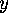is positive. This is the upper half-plane. The prime meridian projects onto the line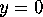to which we have added the point at infinity. We think of the image of the prime meridian as the boundary of the upper half-plane. The linecould be referred to as the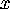axis. We will also refer to it as the real axis,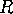.Figure 22: Some h-lines in the upper half-plane.

To define a geometry in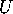we need to define what is meant by a straight line through two points. Given two points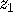and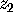in, one can construct many circles passing through both of them since three points determine a circle. However, there is a unique circle passing throughandthat is perpendicular to. We call this circle the straight line passing throughand. When we want to emphasize that we are talking about the hyperbolic line through two points rather than the Euclidean line, we refer to it as an h-line.

You will notice that ifandlie on a vertical line, then there is no Euclidean circle through both that is perpendicular to the boundary. However, recall that a circle on the sphere that passes through the north pole projects onto a what at first glance looks like a line, but is upon reflection can be viewed as a circle (it passes through infinity where it closes up.). We shall also view this line as a circle.

Thus an h-line is either a circle perpendicular to the real axis or a vertical line (see figure 22). (The latter is also automatically perpendicular to the real axis.)

(As a homework exercise you can remind yourself that any circle that intersects the real axis at right angles has its center on the real axis.)

We note that two Euclidean circles are either disjoint, intersect in a point, or intersect in two points. Two circles whose centers are on the real axis that intersect in two points have one point of intersection above the real axis and one below. Thus they have only one point of intersection in the upper half-plane. Similarly, a circle with center on the real axis and a vertical line can have at most one point of intersection in the upper half-plane. Thus any two h-lines are either disjoint or intersect in a point. We have now proved that this system of lines and points satsifies two of the axioms for a geometry.Figure 23: Several h-lines through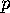that are disjoint from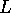.

Discussion

• What does a hyperbolic mirror look like?
• What does a hyperbolic mirror string look like?
• What is the maximum number of mirrors in a Euclidean mirror string?
• What is the maximum number of mirrors in a hyperbolic mirror string?
We turn to the parallel axiom. Again letbe any h-line. For the sake of simplicity assume thatis not a vertical h-line. Letbe any point not on. We can construct a whole family of Euclidean circles whose centers are on the real axis which pass throughand which do not intersect. Figure 23 illustrates several such h-lines. For homework, you can work out either one example or a detailed proof.

Distance

We have emphasized that one of the main distinctions between geometry and topology is that distance is intrinsic to geometry. Thus it behooves us to define a distance in the hyperbolic plane. Again, our emphasis should not be on computing distance, but on having a feel for hyperbolic distance. The important fact to remember is:

• Line segments that appear to be of very different lengths to our Euclidean eyes may be of the same length when we wear hyperbolic glasses and vice-versa.

Next: Distance recipe Up: Geometry and the Imagination Previous: Positive and negative

Peter Doyle